• ## MATLAB之卷积

千次阅读 2019-04-14 10:35:42
文章目录卷积相关卷积定理卷积与互相关，自相关函数的关系卷积原理卷积矩阵表达形式基于MATLAB的不用卷积函数conv求卷积的三种方法 卷积 输入信号：x，系统响应h，输出信号y 卷积：conv(x,h)=y或conv(h,x)=y ...
文章目录卷积的物理意义相关卷积定理卷积与互相关，自相关函数的关系卷积与单位脉冲响应的关系卷积的计算卷积向量表示形式卷积矩阵表达形式基于法一，用MATLAB求X矩阵的三种方法线性系统三个参数，已知两个求另外一个
卷积的物理意义
输入信号通过一个新兴因果移不变系统后的输出为信号与该系统的冲击响应的卷积。任何一个信号都可以表示为单位冲击信号之和。
相关卷积定理
卷积与互相关，自相关函数的关系
条件：假设系统的输入，输出，和单位脉冲响应分别为x(n), y(n), h(n).
Rxy(m) = Rxx(m)*h(m)
对上式进行傅里叶变换，得：
Sxy(w) = Sxx(w)*H(jw)
卷积与单位脉冲响应的关系
x(t)*δ(t) = x(t)
x(t)*δ(t-t0) = x(t-t0)
卷积的计算
卷积向量表示形式
一方不动，另一方前后倒置，从左向右移动，每次移动后重叠部分的乘积，再组合起来即输出信号
                  h1  h2  h3
x4  x3  x2  x1

已知：
输入信号：x=[x1,x2,x3,x4]                  维度：4
系统响应：h=[h1,h2,h3]                      维度：3
输出信号：y=[y1,y2,y3,y4,y5,y6]         维度：4+3-1=6
其中：
y1=x1*h1
y2=x1*h2+x2*h1
y3=x1*h3+x2*h2+x3*h1
y4=x2*h3+x3*h2+x4*h1
y5=x3*h3+x4*h2
y6=x4*h3

卷积矩阵表达形式
将上式写成矩阵形式：
法一：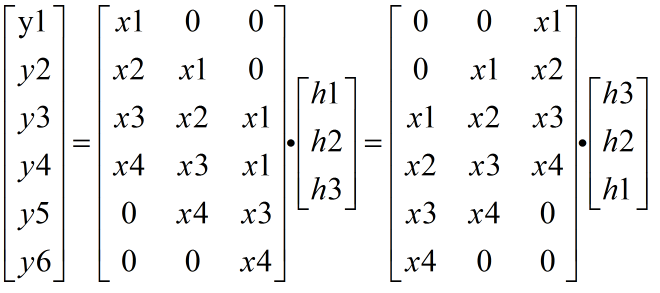法二  ：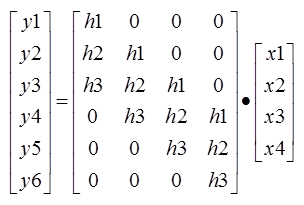基于法一，用MATLAB求X矩阵的三种方法

% 功能：用上述的法一用三种方法求X大矩阵

% 编辑者：lily
% 日期：2019,4,14

clear;
clc;
close all;
% ======================= input signal ==========================
x=[1,2,3,4];
h=[5,6,7];
%求矩阵的向量个数
xn=size(x,2);%求矩阵列数，即几个
hn=size(h,2);
% ============================ 方法1 ====================================
% 原理:按列放
X=zeros(xn+hn-1, hn);%初始化大矩阵

% 观察列的补0规律，求大矩阵X
for  ind=1:hn
xi= [zeros(hn-ind,1);x';zeros(ind-1,1)];
X(:,ind)=xi;
end

Y_noconv=X*rot90(h);
Y_noconv = Y_noconv';
conv=conv(x,h);
% ============================ 方法2 ====================================
% 原理:元素一个一个放
X=zeros(xn+hn-1, hn);%初始化大矩阵
for c = hn:-1:1       % 换列
s = hn - c + 1;     % 从第几行开始放
m = 1;
for r = s:s + xn - 1 % 换行放元素
X(r,c) = x(m);
m = m + 1;
end
end
% ============================ 方法3 ====================================
% 原理：circshift(x, A).将列矩阵往下移动A个数，将其挪到上面

%第一列的0的个数
Mzeros=zeros(1, hn-1);
%第一列元素
Xvec=[Mzeros, x]';
%构造大矩阵
Xmat = [Xvec];
for c = 1:hn-1
Xvec_shift = circshift(Xvec, -c);
Xmat = [Xmat,Xvec_shift];
end


线性系统三个参数，已知两个求另外一个
条件：假设系统的输入，输出，和单位脉冲响应分别为x(n), y(n), h(n).
% 功能：输入信号：x，系统响应h，输出信号y。根据两个求一个
% 用conv，deconv函数，或者写出大矩阵，用矩阵乘法求解

% 编辑者：lily
% 日期：2019,4,14

clear;
clc;
close all;
% ============================ 已知x,h,求y ====================================

x=[1,2,3,4];
h=[5,6,7];
y = conv(x,h);  % or y = conv(h,x);

% ============================ 已知x,y,求h ====================================
h1 = deconv(y,x);
% ============================ 已知h,y,求x ====================================
x1 = deconv(y,h);



展开全文• 原文地址: ... 通过使用卷积定理和MATLAB符号函数, 便可以计算连续函数卷积表达式. 本文主要包括如下几个部分: 1. 利用符号函数计算Fourier变换和Fourier变换 2. 利用符号函数进行卷积计算...
原文地址: https://www.computationalimaging.cn/2018/11/matlab.html
卷积计算起来较为繁琐, 若能够用matlab辅助计算则会简单很多. 通过使用卷积定理和MATLAB符号函数, 便可以计算连续函数的卷积表达式.

本文主要包括如下几个部分:

1. 利用符号函数计算Fourier变换和Fourier反变换

2. 利用符号函数进行卷积计算

3. 以信号与系统考研题中的例题为例进行说明

1. 利用符号函数计算Fourier变换和Fourier反变换

Matlab提供了fourier 和 ifourier 两个内置函数.

下面是fourier的帮助

fourier Fourier integral transform.
F = fourier(f) is the Fourier transform of the symbolic expression
or function f with default independent variable x. If f does not
contain x, then the default variable is determined by SYMVAR.
By default, the result F is a function of w. If f = f(w), then F
is returned as a function of the variable v, F = F(v).

By definition, F(w) = c*int(f(x)*exp(s*i*w*x),x,-inf,inf).

You can set the parameters c,s to any numeric or symbolic values
by setting the preference SYMPREF('FourierParameters',[c,s]).
By default, the values are c = 1 and s = -1.

F = fourier(f,v) returns F as a function of the variable v
instead of the default variable w:
F(v) = c*int(f(x)*exp(s*i*v*x),x,-inf,inf).

F = fourier(f,u,v) treats f as a function of the variable u instead
of the default variable x:
F(v) = c*int(f(u)*exp(s*i*v*u),u,-inf,inf).

Examples:
syms t v w x f(x)
fourier(1/t)   returns   -pi*sign(w)*1i
fourier(exp(-x^2),x,t)   returns   pi^(1/2)*exp(-t^2/4)
fourier(exp(-t)*heaviside(t),v)   returns   1/(1+v*1i)
fourier(diff(f(x)),x,w)   returns   w*fourier(f(x),x,w)*1i

以及ifourier的帮助

ifourier Inverse Fourier integral transform.
f = ifourier(F) is the inverse Fourier transform of the symbolic
expression or function F with default independent variable w. If
F does not contain w, then the default variable is determined by
SYMVAR. By default, the result f is a function of x.  If F = F(x),
then f is returned as a function of the variable t, f = f(t).

By definition,
f(x) = abs(s)/(2*pi*c) * int(F(w)*exp(-s*i*w*x),w,-inf,inf).

You can set the parameters c,s to any numeric or symbolic values
by setting the preference SYMPREF('FourierParameters',[c,s]).
By default, the values are c = 1 and s = -1.

f = ifourier(F,u) returns f as a function of the variable u
instead of the default variable x:
f(u) = abs(s)/(2*pi*c) * int(F(w)*exp(-s*i*w*u),w,-inf,inf).

f = ifourier(F,v,u) treats F as a function of the variable v
instead of the default variable w:
f(u) = abs(s)/(2*pi*c) * int(F(v)*exp(-s*i*v*u),v,-inf,inf).

Examples:
syms t u v w f(x)
ifourier(w*exp(-3*w)*heaviside(w))  returns  1/(2*pi*(-3+x*1i)^2)
ifourier(1/(1 + w^2),u)   returns   exp(-abs(u))/2
ifourier(v/(1 + w^2),v,u)   returns   -(dirac(1,u)*1i)/(w^2+1)
ifourier(fourier(f(x),x,w),w,x)   returns   f(x)

显然, 有了这两个函数, 我们便可以利用符号变量计算Fourier变换和反变换.

例如, 对于奥本海姆<信号与系统(第2版)>习题4.1(b)

计算$f(t)=e^{-2|t-1|}$的傅里叶变换

我们便可利用MATLAB进行计算:

syms t
fourier(exp(-2*abs(t-1)))

得到如下结果:

>> fourier(exp(-2*abs(t-1)))

ans =

- exp(-w*1i)/(- 2 + w*1i) + exp(-w*1i)/(2 + w*1i)

本题的参考答案为:对比可知, 通过MATLAB得到的结果与实际结果相同.

同理, 我们也可以计算Fourier的反变换. 如下所示

>> syms w
>> ifourier(dirac(w))

ans =

1/(2*pi)

2. 利用符号函数进行卷积计算

通过计算Fourier变换和反变换, 我们便可以结合卷积定理, 如式\ref{eq1}所示, 求出任意两个函数的卷积.

$$x(t)*h(t)=F^{-1}(X(jw)\dot H(jw)) \tag{1} \label{eq1}$$

例如, 我们用$x(t)=e^{cos(3t+\frac{1}{4}\pi)}$与$\delta(t-3)$的卷积进行验证, 有dirac函数的性质, 结果显然应为$x(t)=e^{cos(3(t-3)+\frac{1}{4}\pi)}$

syms t
>> xt = exp((cos(3*t + 0.25*pi)))

xt =

exp(cos(3*t + pi/4))

>> f_xt=fourier(xt)

f_xt =

fourier(exp(cos(3*t + pi/4)), t, w)

>> f_ht=fourier(dirac(t-3))

f_ht =

exp(-w*3i)

>> result = ifourier(f_xt * f_ht)

result =

exp(cos(3*x + pi/4 - 9))

结果正确.

3. 以信号与系统考研题中的例题为例进行说明

题目为:

若$x(t)=\frac{sint}{t}$, 则积分$\int_{-\pi}^{\pi}[x^2(t)*sin(t+\frac{\pi}{3})]dt$的值为多少?

正解为0, 方法是求出$x^2(t)$和$sin(t+\frac{\pi}{3})$的Fourier变换, 通过卷积定理, 再利用三角函数的周期性即可得到结果为0. (欢迎补充更简单的方法~)

用MATLAB则可通过如下代码求解:

syms t
ft = sin(t)*sin(t)/(t*t);
ft = sin(t)*sin(t)/(t*t);
vt = sin(t+pi/3);
f_ft = fourier(ft);
f_vt = fourier(vt);
f_anss = f_ft * f_vt;
fv = ifourier(f_anss);
result = int(fv, [-pi, pi])

输出为:

result =

0

可见, 利用MATLAB能够比较好地计算连续函数卷积结果的表达式, 并可以用来解决实际的问题. 其中, dirac函数, li表示的虚部, 以及fourier和ifourier函数都是之前没用过的新函数.


展开全文信号与系统
• 利用MATLAB里的反卷积和反池化实现 王同学，想请你帮我思考一下做个东西：不知道你看过那个visualizing and understanding cnn那篇文章没有。你可以看到，他的可视化是通过上采样以及反卷积把某层的冲激响应映射会...
• 修复反色反卷积函数中的对比度缩放（RecombineStains） 有关颜色反卷积的更多资源 斐济实施说明 python scikit-image实现 斐济实施的深入说明 Antony Chan的另一个Matlab实现（由于两个嵌套的for循环而变慢） Matlab...
• 这是一个基于matlab图像复原处理包，其中包括图像的模糊、维纳滤波、盲反卷积、点扩散函数和光学转换函数互相转化
• 使用反卷积函数通过20次迭代来估算44个宽视野[WF]（观察/模糊），44个结构化照明[SIM]（地面真实情况）PSF。 更多的迭代可能会带来更好的结果，但如果这样，请使用edgetaper，否则可能会产生振铃效果。 3.阅读提示...
• 在应用 od AR 滤波器后，信号通过最小熵反卷积。 这种组合的 AR+MED 方法带出了隐藏在噪声中的轴承故障。 该函数为单独的 AR 绘制两个图形，为 AR+MED 绘制另一个图形例子： 负载（'s4.mat'）; 信号=s4; Fs=12000...
• 函数非常快速地将一个非常大的向量从另一个非常大的向量中解卷积。 速度的巨大提升是由于使用了两个 fft 和一个 ifft。 要了解如何使用此程序，请将其放在您的“工作”文件夹中并键入“help fdeconv”。
• 对于浊音部分，我们的喉咙就像一个传递函数。 元音包含在此类别中。 清音部分描述了语音中类似噪声的声音。 这些是用我们的嘴和舌头（而不是我们的喉咙）发出的声音，例如“f”音、“s”音和“th”音。 通常在计算...
• 最小熵解卷积 (MED) 滤波器用于强调信号的脉冲部分。 它被开发用于处理地震数据，但已广泛用于处理轴承和齿轮退化是一个问题的机械信号。 此函数采用单个向量 (x)、滤波器长度 (L) 和最大迭代次数 (maxIter)，并迭代...
• 盲解卷积是指在不确切了解卷积中使用的脉冲响应函数的情况下对信号进行解卷积。 这通常是通过对输入和/或脉冲响应添加适当的假设来恢复输出来实现的。 我们在这里考虑输入信号的稀疏性或简约性。 它通常用 l0 成本...
• Lsqnonlin代码用于MS2数据的反卷积，多指数回归和模型推断包 版本：2021年4月 蒙彼利埃大学（University of Montpellier）的奥维迪乌·拉杜列斯库（Ovidiu Radulescu）撰写版权：本出版物以3节BSD发表 该软件包包含...
• GPU使用CUDA加速了3D图像反卷积。 由Lin Shao和Dan Milkie在Janelia的Betzig实验室开发。 笔记 在此“ C：\ cudaDecon \ CMakeLists.txt”中指定了兼容的GPU。 这也设置了所有到依赖库的链接。 如果最终添加其他代码...
• 为了快速、准确地在轨估算空间光学遥感成像系统的点扩展函数(PSF)，提出了基于多通道盲反卷积（MBD）的估算方法，避免了以往方法依赖靶标及时间开销大等缺点。从单幅遥感图像中，提取多幅具有局部一致背景的目标子...
• 为了克服这个限制，我们在这里提出一种盲反卷积方法，该方法能够从多普勒数据中估计血液成分和PSF。 与基于实验测量的PSF的先前方法和其他两种最新方法相比，对模拟和体内数据进行的数值实验从定性和定量方面证明了...
• 函数绘制高斯脉冲响应、单位脉冲和使用 MATLAB 的“过滤器”对两者进行 % 卷积/反卷积。 具体来说% 它使用 'filter' 而不是 'deconv' 来进行反卷积，从而％返回原始的高斯向量以及单位响应向量。 % 向量长度在所有...
• matlab函数大全-matlab函数大全.doc 比较白痴的东西，不过对初学者也许有用，特分享一下。不要喷我哦!函数具体用法可以用help查一下。附件内容与下面一样的。 Aabs 绝对值、模、字符的ASCII码值 acos 余弦 ...
• - 执行反卷积函数 -voigtNormGLform.m-创建与最大强度1对应的Voigt轮廓函数，定义宽度和位置 - complexErrorFunction.m 和 complexErrorFunction.m 计算由 F. Schreier 博士友情提供的复杂误差函数和 Voigt 配置...
• 研究波传播的鲁棒反卷积函数。 低通滤波和将输入信号重新采样到更高的采样率可能有助于消除噪声并改善拾取峰值。 zip 文件中包含一个示例 MatLAB 例程，该例程具有实际输入信号以复制此处显示的绘图。
• 卷积、高斯滤波、直方图均衡化、拉普拉斯滤波、形态学滤波、傅里叶变换等。
• 一种是基于FFT的卷积（fftConvolutionTest），另一种是基于FFT的反卷积（fftDeconvolutionTest）。 第一个演示可以通过高斯核和圆核对图像进行卷积处理。 Seconde演示可以使用普通或Weiner滤波器对高斯模糊进行...
• Matlab使用初步及信号描述与变换9.1 Matlab使用初步及信号描述...信号运算有：信号的叠加与相乘、信号的褶、信号的时间平移、信号的时间展缩、卷积运算等。(2)编写程序syms t;f1 = sym('3*sin(pi / 2 * t)');subp...
Matlab使用初步及信号描述与变换9.1 Matlab使用初步及信号描述与变换(1)弄清原理，说明信号描述、信号运算及变换方法。描述信号的基本方法是写出它的数学表达式，该表达式是一个或若干个自变量的函数或序列的形式。信号运算有：信号的叠加与相乘、信号的反褶、信号的时间平移、信号的时间展缩、卷积运算等。(2)编写程序syms t;f1 = sym('3*sin(pi / 2 * t)');subplot(4,3,1),ezplot(f1,[-5,5]),grid onf2 = sym('3*sin(pi * t)');subplot(4,3,2),ezplot(f2,[-5,5]),grid onf3 = sym('3*sin(3 * pi / 2 * t)');subplot(4,3,3),ezplot(f3,[-5,5]),grid onf4 = subs(f1,t,-t);subplot(4,3,4),ezplot(f4,[-5,5]),grid onf5 = subs(f2,t,-t);subplot(4,3,5),ezplot(f5,[-5,5]),grid onf6 = subs(f3,t,-t);subplot(4,3,6),ezplot(f6,[-5,5]),grid onf7 = subs(f1,t,t-2);subplot(4,3,7),ezplot(f7,[-5,5]),grid onf8 = subs(f2,t,t-2);subplot(4,3,8),ezplot(f8,[-5,5]),grid onf9 = subs(f3,t,t-2);subplot(4,3,9),ezplot(f9,[-5,5]),grid onf10 = subs(f1,t,1-2*t);subplot(4,3,10),ezplot(f10,[-5,5]),grid onf11 = subs(f2,t,1-2*t);subplot(4,3,11),ezplot(f11,[-5,5]),grid onf12 = subs(f3,t,1-2*t);
展开全文• 3. 掌握序列的相加、相乘、移位、褶等基本运算及计算机实现与作用 4. 掌握线性卷积软件实现的方法 5. 掌握计算机的使用方法和常用系统软件及应用软件的使用 6. 通过编程，上机调试程序，进一步增强使用MATLAB的...
实验目的和要求
1.  掌握各种常用的序列，理解数学表达式和波形表示
2.  掌握在计算机中生成及绘制数字信号波形的方法
3.  掌握序列的相加、相乘、移位、反褶等基本运算及计算机实现与作用
4.  掌握线性卷积软件实现的方法
5.  掌握计算机的使用方法和常用系统软件及应用软件的使用
6.  通过编程，上机调试程序，进一步增强使用MATLAB的能力

实验内容和原理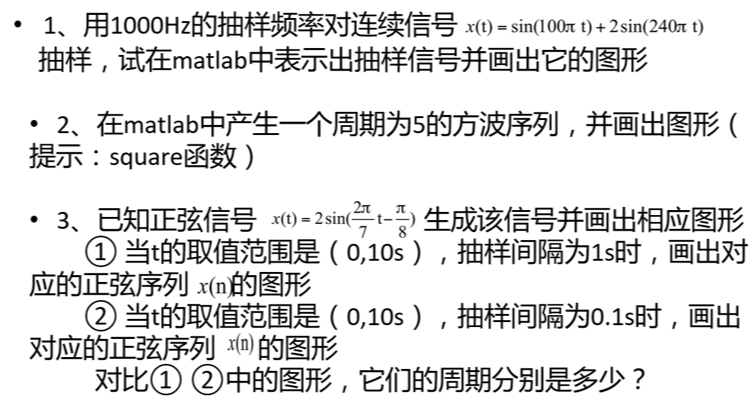主要仪器设备
装有MATLAB的计算机一台
操作方法和实验步骤
1.根据课堂展示过的范例，更改采样频率为100Hz，采样信号重新设定，在MATLAB中输入指令如下即可得到采样图形。
直接在命令行中运行结果如下：

>> fs=1000;
>> t=0:1/fs:1;
>> x=sin(100*pi*t)+2*sin(240*pi*t);
>> stem(t(1:200),x(1:200),'filled');
>> axis([0 0.1 -3 3])


查看并保存图像。

2.要得到方波序列，可以先利用square（T）函数产生方波，在对其进行采样得到对应的方波序列，在MATLAB里输入help square 即可查看函数的使用方法，了解到它的周期和sin一样是2*pi，要使周期为5,则参数应该定为0.4*pi，写出代码如下：

>> fs=1;
>> t=0:1/fs:10;
>> x=square(0.4*pi*t);
>> stem(t,x,'filled')


查看并保存图像。
3.由plot函数可以画出连续信号，根据题目要求可以写出如下代码：

>> x=2*sin(2*pi/7*t-pi/8);
>> plot(t,x);
>> title('初始信号');
>> xlabel('t')


查看并保存图像。
（1）根据题中所给取值范围和采样间隔，可以知道t从0开始到10，采样频率为1Hz，则可以写出如下代码：

>> t=0:1:10;
>> x=2*sin(2*pi/7*t-pi/8);
>> stem(t,x,'filled')


查看并保存图像。
2）同理可知，t从0到10，采样频率为10Hz，则代码如下：

>> t=0:0.1:10;
>> x=2*sin(2*pi/7*t-pi/8);
>> stem(t,x,'filled')


查看并保存图像.

实验结果与分析
1.运行代码后可以得到图像如下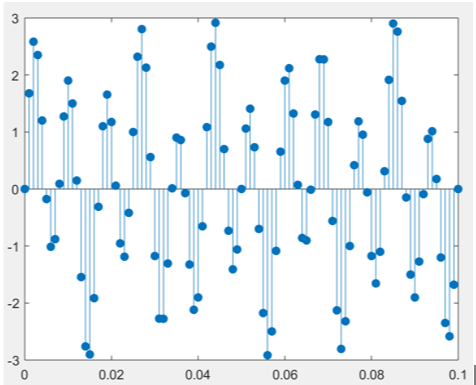2.运行代码后得到图像如下：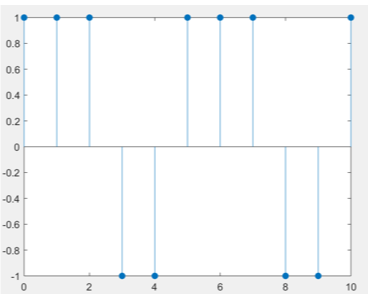可见其为周期性的方波序列。
3.根据表达式用plot函数得到图像如下：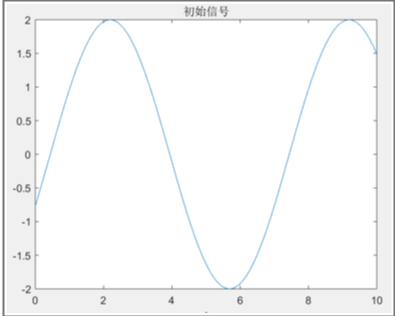以1Hz采样频率采样得到的序列如下：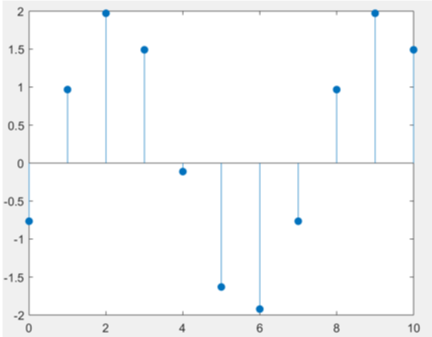目测大致也是可以看出轮廓为正弦函数，可见周期为7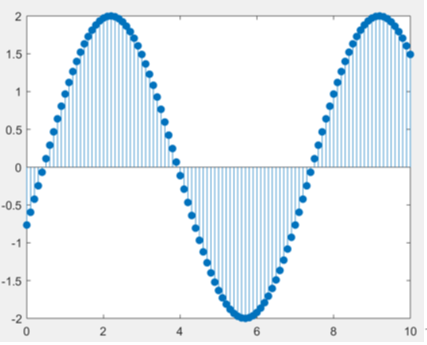十分清晰的轮廓，没有任何困难就可以辨认出是正弦图像，由图像可见周期为7
注：
1.  由于实验在MATLAB中进行，几乎不需要成本，所以可以尽可能多的多做尝试，充分了解MATLAB的指令语言以及功能。
2.  使用分号结尾可以暂时不显示代码运行结果，最后采用回车即可得到最终结果，使得代码之间较为紧凑，输入也可以变得更快。
展开全文• 傅里叶变换函数fft函数：fftshift函数：ifft函数：傅里叶变换 3.滤波器分析与实现函数conv函数：完成向量的卷积操作。impz函数：产生系统的冲击响应。zplane函数：绘制系统的零极点图。 abs函数：计算向量的...工具 filter fft
• 要在最终的非盲反卷积步骤中运行完整算法，您还需要下载ICCV 2011论文“从自然图像斑块的学习模型到整个图像恢复”中描述的EPLL方法的实现，作者是Daniel Zoran和Yair Weiss，并将其添加到您的MATLAB路径。 可以从...
• 去噪声代码matlab 群体内部和群体之间的稀疏性 Matlab代码与I.Bayram和S.Bulek于2017年发表的论文《促进群体内和群体间稀疏性的惩罚功能》一起使用...（3）反卷积实验：主文件是文件夹“反卷积”中的“ DemoDeconv.m”
• 冲激响应：叠加和反卷积 排序后的傅立叶变换信号被发送到simdecf.m中，以解卷积为类似于Greens函数的形式。 它们保存在rft ，通过逆傅立叶变换传递，以恢复rec 。 过滤脉冲响应，获取数据tps 使用带通滤波器(0.04, 1
• 这个文件是一个例子，展示了如何测试四阶自动反卷积函数：autodeconv.m
• matlab参数建模法代码OASIS：钙成像数据的在线快速反卷积 随附文件“”的代码。 Python： MATLAB： 开始使用 安装 将OASIS函数添加到MATLAB的搜索路径 >> oasis_setup 处理yoru数据 还有一个高级函数deconvolveCa.m ...
• 数字信号处理——基于计算机的方法（一）第二章 时域中的离散时间信号集合平均卷积复数...可以使用matlab中的卷积函数conv() 运行结果： 复数序列的共轭（）对称 运行结果： 因此可以看出，共轭对称序列的实部数字信号处理# matlab反卷积函数matlab 订阅